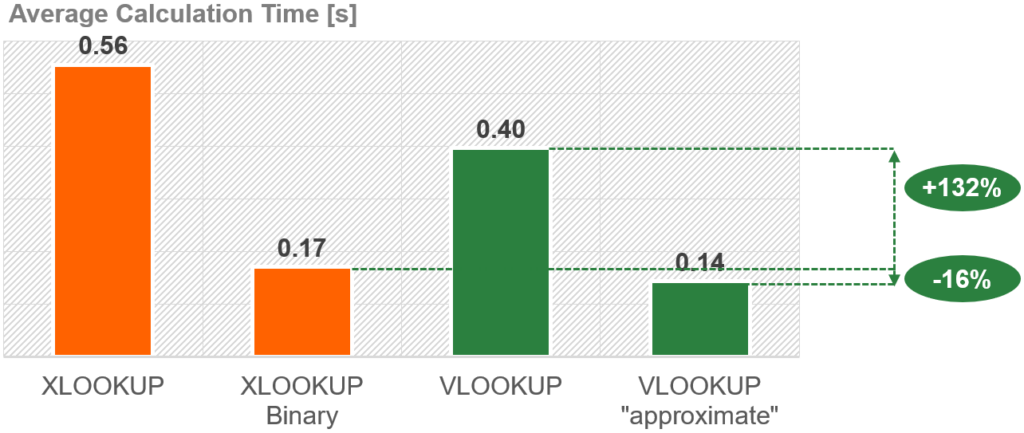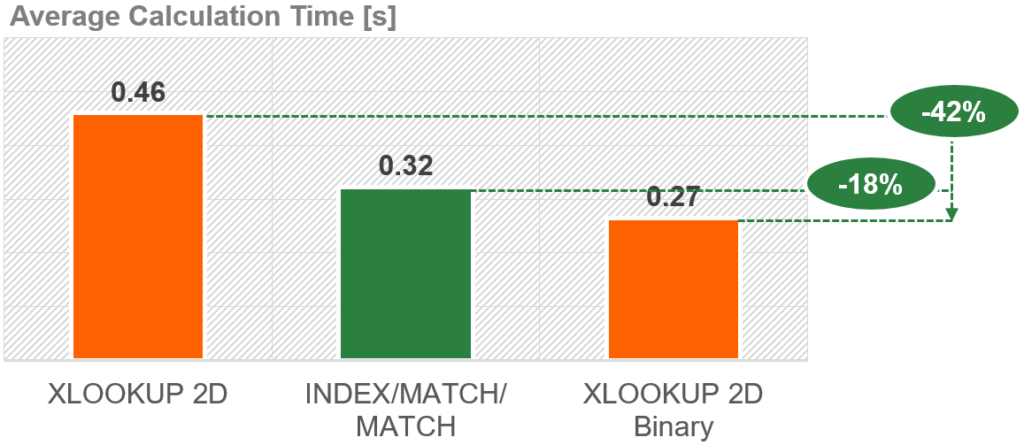# Performance of XLOOKUP: How fast is the new XLOOKUP vs. VLOOKUP?

We’ve explored the basics of XLOOKUP, discovered a wide range of advanced use cases for XLOOKUP and the advantages towards VLOOKUP and INDEX/MATCH. What we have not talked about so far is the performance of XLOOKUP. Especially for larger Excel models with possibly hundreds of thousands of functions to calculate, performance is a major concern. Let’s see how XLOOKUP performs vs. VLOOKUP and INDEX/MATCH!

## Introduction

As I am often working with large Excel models, I’m always concerned about performance. I have already published a book about how to increase the performance of Excel files and written several articles:

So, as you can see, I’m pretty interested in the performance topics. Knowing a few basics can really save a lot of time here. That’s why I’ve taken a close look at the XLOOKUP function. How fast is it compared to VLOOKUP? Or INDEX/MATCH?

## Methodology

As again for my previous articles, my goal is not to have some laboratory results. I rather want to know, how Excel behaves under realistic work conditions.

• Measuring is done with an Excel file with 100,000 XLOOKUP functions (and again with the same number of VLOOKUP and INDEX/MATCH functions for the same lookup).
• A VBA Macro determines the exact calculation time.
• The test is done on a MacBook Air running Windows vis Bootcamp. That’s probably the low end of performance which should probably be more realistic than using the newest, fastest PC on steroids… 😉
• Each setting is calculated 20 times. All runs where regarded.

## Results

### Performance of XLOOKUP vs. VLOOKUP

The most pressing question: How fast is XLOOKUP vs. VLOOKUP? Is XLOOKUP really an alternative for VLOOKUP in terms of calculation speed?

Surprisingly, XLOOKUP needs ~40% longer to calculate than VLOOKUP in our example file.

So, should you avoid XLOOKUP now? Hold on, not that fast… XLOOKUP offers much more built-in options, including a “binary” search mode. We’ll come to that later.
Also, we’ve seen many great use cases for XLOOKUP. And further considering that the absolute calculation time on a low end computer is still fast: roughly half a second for 100,000 XLOOKUPS is usually not considered bad.

### Performance of XLOOKUP vs. INDEX/MATCH

So, knowing that XLOOKUP is slower than VLOOKUP is fine by itself. But many Excel users (including me) use INDEX/MATCH much more often for lookups. How is the performance of XLOOKUP vs. INDEX/MATCH?

As you can see in the chart on the right-hand side, the calculation time for INDEX/MATCH is pretty much the same as for VLOOKUP. Because calculation times for VLOOKUP and INDEX/MATCH are on a similar level, the performance of XLOOKUP compared to INDEX/MATCH doesn’t surprise much: XLOOKUP is significantly slower than INDEX/MATCH as well.

But more: Excel also has a new XMATCH function. So, what about the performance of XLOOKUP vs. INDEX/XMATCH?

XLOOKUP is slightly faster than INDEX/XMATCH. That leads to the conclusion: All new “X-functions” in Excel are slower than their traditional counterpart.

### Calculation time of Binary XLOOKUP vs. normal XLOOKUP

Now it get’s really interesting. We know that XLOOKUP is slower than VLOOKUP and INDEX/MATCH. But the previous results are only considering “normal” lookups, not utilizing the built-in binary XLOOKUP search mode.

#### What is the “binary search mode” of XLOOKUP?

A binary search is the way, Excel (or any other program) searches for a value. It relies on sorted data: Instead of looping through all items in a list of table top-down, it cuts it into half. If the search value is smaller then the value at the half / median, it divides the lower half of the values into half again and so on. Wikipedia has more infomation about binary searches.

The big advantage: Binary searches or usually much faster than “linear” searches.

The disadvantage: The data must be sorted.

#### So, how fast is a binary XLOOKUP vs. a normal XLOOKUP?That’s impressive: the binary XLOOKUP can save 69% of calculation time compared to a normal XLOOKUP.

That’s impressive: In our example, 69% of the calculation time can be saved using a binary XLOOKUP compared to a normal XLOOKUP. That means in absolute times, the test case just needed 0.17s to calculate 100,000 XLOOKUP functions.

When we compare it to the normal VLOOKUP functions (0.40s on average for 100,000 VLOOKUPs; see above), you still save more than half the time.

#### Performance of a binary XLOOKUP vs. VLOOKUP with “approximate match”

As you may know – or have been wondering – also the VLOOKUP function has such “high-speed” mode. It works pretty much the same way. If you put “TRUE” as the last argument of VLOOKUP, VLOOKUP searches for an “approximate match”. So, how does a binary XLOOKUP compare to a “approximate match” VLOOKUP?Compared to a normal VLOOKUP, the binary XLOOKUP is significantly faster. But a VLOOKUP with a approximate match is still a little bit faster.

The binary XLOOKUP is slightly slower than an approximate VLOOKUP (~16% slower). But compared to both other options (normal XLOOKUP or normal VLOOKUP), the binary XLOOKUP is significantly faster.

### 2D XLOOKUP vs. INDEX/MATCH/MATCH

For the following comparison I’ve used a fairly simple version of two XLOOKUP nested into each other (please refer to this article for more information). Also, for INDEX/MATCH/MATCH, the function I’ve used was in it’s simplest form.

We’ve seen that INDEX/MATCH is much faster than XLOOKUP. The same seems to be true for INDEX/MATCH/MATCH in comparison with a 2D XLOOKUP. INDEX/MATCH/MATCH calculates around 30% faster than a 2D XLOOKUP in our test workbook.

### “Normal” 2D XLOOKUP vs. Binary 2D XLOOKUP

This is our last test examining the performance of XLOOKUP. How much time can we save if we use a binary 2D XLOOKUP instead of a normal 2D XLOOKUP?But again, the performance of a binary 2D XLOOKUP is better than a “normal” 2D XLOOKUP and INDEX/MATCH/MATCH.

As expected, a 2D XLOOKUP with a binary search mode is much faster than a normal 2D XLOOKUP. It also needs 18% less time to calculate than an INDEX/MATCH/MATCH.

This comparison was inspired by the comments below (thanks, Øystein and Johan!).

Because the XLOOKUP function has a built-in “Not Found” argument, the question is if it’s faster to use this or wrap a traditional IFERROR function around XLOOKUP (and around VLOOKUP). Here are the results:

Legend by numbers:

The first three columns (1-3) never returned an error. That means, all values were found.

1. XLOOKUP function using the “If Not Found” argument; no error and all values were found.
2. IFERROR function wrapped around the XLOOKUP function; again, all values found (no error).
3. Same as number two but using the VLOOKUP instead of the XLOOKUP function.

The first two options (XLOOKUP with “If Not Found” argument vs. IFERROR around XLOOKUP) need similar calculation times (~6% difference but due to the test setup probably statistically not significant). But to using the “traditional” IFERROR around the VLOOKUP function is significantly faster.

The next three columns (4-6) compare if all function return #N/A errors.

2. IFERROR function wrapped around the XLOOKUP function; all functions return #N/A errors and therefore jump to the second argument of the enclosing IFERROR function.
3. Same as number two but using the VLOOKUP instead of the XLOOKUP function.

Also in this case (when all XLOOKUPs and VLOOKUPs would lead to #N/A errors), the two XLOOKUP versions (numbers 4 and 5) have similar results. But when it comes to the traditional IFERROR with the VLOOKUP function, the difference is quite big: calculation times seem to significantly lower with the IFERROR / VLOOKUP combination.

Do you want to boost your productivity in Excel?

Get the Professor Excel ribbon!

Add more than 120 great features to Excel!

## Summary of the performance of XLOOKUP

XLOOKUP is much more powerful than VLOOKUP or INDEX/MATCH. It offers a great range of options right built-in. But when it comes to performance, XLOOKUP is significantly slower than it’s “old” competitors VLOOKUP and INDEX/MATCH. The binary search mode definitely helps here. Also, the results for a 2-way-lookup are similar: a “normal” 2D XLOOKUP is slower than INDEX/MATCH/MATCH, but a binary 2D XLOOKUP can save a lot of time.Henrik Schiffner is a freelance business consultant and software developer. He lives and works in Hamburg, Germany. Besides being an Excel enthusiast he loves photography and sports.

1.Øystein Dahl says:

I was waiting for a check on iferror(vlookup()) vs xlookup() with use of the built in “if error”

1.Johan says:

From my initial testing, wrapping the xlookup in ‘iferror’, or using xlookup’s built in ‘if error’ parameter delivers almost identical performance for both approaches. This implies that xlookup’s built in ‘if error’ parameter is about as efficient as the standalone iferror function. As a result, the relative performance of the tests in this article should be the same weather you wrap vlookup, index/match or xlookup in the iferror function, or if you use xlookups built-in iferror parameter

1.Henrik Schiffner says:

Hi Øystein, hi Johan,

Thanks for the questions and feedback. I have updated the article including a comparison of XLOOKUP with the “If Not Found” argument vs. IFERROR(XLOOKUP) and IFERROR(VLOOKUP).

Are these results in line with your experience?

2.Nahcr says:

i had a spreadsheet with xlookups that slowed to an unbearable crawl. going back to vlookups solved the problem. your iferror comparison also suggests that using iferror as a lazy persons final choice may not be ideal if it is possible to select out some error rows with a prior if: (if(expectedNotFound),doThis,iferror(vlookup(),unexpectedNotFoundDoThis. its a pity because vlookups also return annoying 0 if there is a blank cell, whereas xlookup returns blanks for blank cells. And each vlookup has to have the looked up column number manually inserted, whereas xlookup allows you to copypaste xlookups across columns so that the looked up columns are auto generated (as long as they follow each other in the lookup table). but speed is more important

3.Alan says:

Just what I was looking for with the speed comparison -is there any consideration of the memory used for each function?
For example, lookup requires the whole table array, whereas lookup requires just the 2 relevant column arrays
Thanks

4.Niki says:

Hi,

I’m struggling to find the right formula to multiply units by rates.

I have different materials with different units and rates are depend on quantities.

I have a more than 2000 row spreadsheet and units also varies that means that the formula also need to find the unit on sheet 1. Rate criteria can also change on sheet 1.

Details on Sheet 1
Unit Rate1(not exceeding) Rate2(not exceeding) Rate3(not exceeding) Rate4(exceeding)
day
h
m (QB) 10 50 200 200
m
m2 (QB) 10 50 150 200
m2

Details on Sheet 2

Description Unit Quantity Rate 1 Rate 2 Rate 3 Rate 4 Price
path m (QB) 9 11 13 13.5 14
road m (QB) 51 5 10 15 20
wall m2 (QB) 35 10 15 20 25
wood m 20 11
paint m2 150 12

And I’m looking for the price.
5.Oliver says:
6.Sa says: